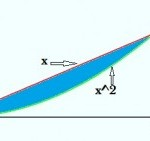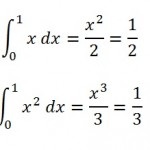# Integrals

## How to find the area between two curves in integral calculus

Finding the area between two curves in integral calculus is a simple task if you are familiar with the rules of integration (see indefinite integral rules above). The easiest way to solve this problem is to find the area under each curve by integration and then subtract one area from the other to find the difference between them. You may be presented with two main problem types. The first is when the limits of integration are given, and the second is where the limits of integration are not given.

## Area Between Two Curves: Limits of Integration Given

Sample problem 1: Find the area between the curves y = x and y = x2 between x = 0 and x = 1.Step 1: Find the definite integral for each equation over the range x = 0 and x = 1, using the usual integration rules to integrate each term. (see: calculating definite integrals).Step 2: Subtract the difference between the areas under the curves. You’ll need to visualize the curves (sketch or graph the curves if you need to); you’ll want to subtract the bottom curve from the top one. The curve on top here is f(x)=x, so:
1213 = 16.

## Limits of Integration NOT Given

Sample problem: Find the area between the curves y = x and y = x2.

Step 1: Graph the equations. In most cases, the limits of integration will be clear, especially if you’re using a TI-calculator with an Intersection feature (just find the intersections of the two graphs). If you can find the intersection by graphing, skip to Step 3.

Step 2: Find the common solutions of these two equations if you cannot find the intersection by graphing (treat them as simultaneous equations).
Substituting y = x for x in y = x2 gives an equation y = y2, which has only two solutions, 0 and 1.
Putting the values back into y = x to give the corresponding values of x: x = 0 when y = 0, and x = 1 when y = 1. The two points of intersection are (0,0) and (1,1).

Step 3: Complete the steps in Sample Problem 1 (limits of integration given) to complete the calculation.